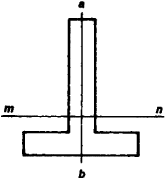# Axis, Neutral

Also found in: Dictionary.
The following article is from The Great Soviet Encyclopedia (1979). It might be outdated or ideologically biased.

## Axis, Neutral

(in strength of materials), for a beam that undergoes bending, a line in a cross section of the beam such that at all points of the line the normal stresses parallel to the axis of the beam are equal to zero. The neutral axis divides the cross section into two parts: the normal stresses are tensile in one part and compressive in the other. If ab (see Figure 1)—the line through which the plane of action of the external forces passes—is one of the principal axes of inertia for the cross section shown in Figure 1, then the neutral axis mn will be the second principal axis. The locus of all the neutral axes forms the neutral surface of a beam.Figure 1